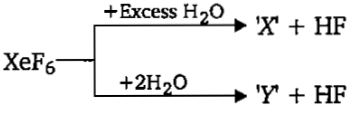${\mathrm{XeF}}_{6}$ dissolves in anhydrous $\mathrm{HF}$ to give a good conducting solution which contains :

High Yielding Test Series + Question Bank - NEET 2020

Difficulty Level:

Which of the following is not true about helium?

(a) It has the lowest boiling point

(b) It has the highest first ionization energy

(c) It can diffuse through rubber and plastic material

(d) It can form clathrate compounds

High Yielding Test Series + Question Bank - NEET 2020

Difficulty Level:

Which of the following is an uncommon hydrolysis product of ?

High Yielding Test Series + Question Bank - NEET 2020

Difficulty Level:

${\mathrm{SbF}}_{5}$ reacts with ${\mathrm{XeF}}_{4}$ to form an adduct. The shapes of cation and anion in the adduct are respectively:

(a) square planar, trigonal bipyramidal        (b) T-shaped, Octahedral

(c) square pyramidal, octahedral                 (d) square planar, octahedral

High Yielding Test Series + Question Bank - NEET 2020

Difficulty Level:

Incorrect statement regarding following reactions is :(a) 'X' is explosive

(b) 'Y' is an oxyacid of xenon

(c) Both are example of non-redox reaction

(d) ${\mathrm{XeF}}_{6}$ can undergo partial hydrolysis

Concept Questions :-

group 18,perparation and properties
High Yielding Test Series + Question Bank - NEET 2020

Difficulty Level:

Which of the following noble gas does not form clathrate compound?

Concept Questions :-

group 18,perparation and properties
High Yielding Test Series + Question Bank - NEET 2020

Difficulty Level:

Correct order of bond angle in given species is :

Concept Questions :-

group 16,preparation and properties
High Yielding Test Series + Question Bank - NEET 2020

Difficulty Level:

The incorrect order is:

Concept Questions :-

group 16,preparation and properties
High Yielding Test Series + Question Bank - NEET 2020

Difficulty Level:

Among the following cyclic species are :

Concept Questions :-

group 15 ,preparation and properties
High Yielding Test Series + Question Bank - NEET 2020

Difficulty Level:

$HCN+{H}_{2}O\to \left[X\right]+N{H}_{3}$

$\left[X\right]\stackrel{∆}{\to }\left[Y\right]+{H}_{2}O$

(Unbalanced equations)

[Y] May be prepared by which one of the following methods?

[A] By dehydration of malonic acid with ${P}_{2}{O}_{5}$

[B] By thermal decomposition of carbon sub-oxide in air.

[C] By heating potassium hyxacyaanoferrate (II) with conic ${H}_{2}S{O}_{4}$

[D] By the action of conc. $HN{O}_{3}$ on charcoal

Concept Questions :-

group 15 ,preparation and properties